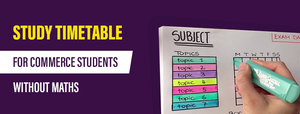RRB NTPC 2020 Syllabus: Mathematics - Study24x7New to Study24X7 ?

# RRB NTPC 2020 Syllabus: Mathematics

Updated on 16 October 2020RRB 2020-21 (Railway Jobs)
Updated on 16 October 2020The Board will soon release the RRB NTPC 2020 notification for aspirants. But the preparation for the RRB-NTPC 2020 exam must be started already.

Mathematics is a major part of the RRB NTPC exam syllabus as it is part of both stages of computer-based tests. The candidates must prepare the topics from the syllabus in detail and keep on practicing on a regular basis to perform well in the examination.

## Tips to solve mathematics paper in RRB NTPC exam:

1. Prepare the individual topics from the syllabus in detail.
2. Learn the shortcuts and tricks to speed up the calculation.
3. Remember all the important formulas, information,  and tables on your fingertips.

## Some of the important topics from RRB NTPC mathematics syllabus

1. Number system: It is a way of writing numbers. Here you need to understand the difference between types of numbers like whole Number, natural number, integers, prime number, etc.
2. Decimals: It is a way of representing the number in arithmetic in the multiple of 10. It is used to express fractions.
3. Fractions: Fractions are terms expressed in the form of p/q. where p is numerator and q is denominator (q≠0).
4. LCM (Least Common Factor): It represents the lowest common number from the factors of a number.
5. HCF (Highest Common Factor): This represents the largest number that divides the two or more numbers.
6. Ratio & Proportion: Ratio is the relation between two or more values of the same
7. kind. It expresses in the form of is antecedent and consequent. For example, X/Y.

Proportion (::) is expressed when the ratio of the first and second elements is equal to

the third and fourth elements.

1. Percentage: Percentage (%) is a fraction or ratio where the denominator is 100.
2. Mensuration: This technique is used to calculate the area, perimeter, and volume of different geometrical structures.
3. Time and work: This topic consists of the solution of problems related to work done at a fixed period of time.  More work can be done at the same time if we deploy more people.
4. Time and distance: The problems related to moving objects are studied under this topic. There are three major observations in the questions related to speed, distance and time. The basic formula used in this topic is Distance= Speed X Time.
5. Simple & Compound Interest: In RRB NTPC 2020 examination, questions may be asked on either simple interest or compound interest.
6. Profit & Loss:The Profit and loss concept is related to buying or selling of particular commodity.
7. Elementary Algebra: This deals with the operations and properties of arithmetic. It is an important field of mathematics.
8. Geometry and Trigonometry: Basics of geometry include different types of structures. Trigonometry is concerned to solve the problems on the right-angle triangle.
9. Elementary Statistics: It deals with the collection, analysis, and interpretation of data.

We hope that this list of important topics from the RRB NTPC 2020 Mathematics Syllabus would be of help to you.

All The Best To All The RRB NTPC 2020 Aspirants !

Write a comment...Trending ArticlesBy CBSE CLASS 8By CBSE CLASS 10By Examination GuideBy K-12-Commerce
Related Posts